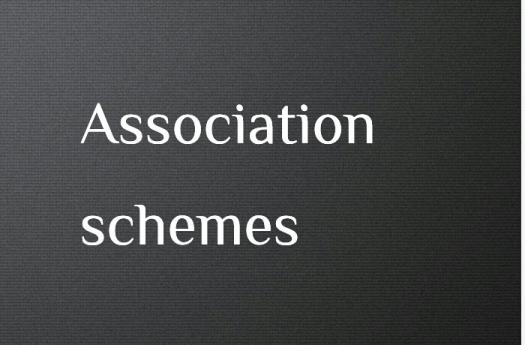# What Do You Know About Association Schemes?

Approved & Edited by ProProfs Editorial Team
At ProProfs Quizzes, our dedicated in-house team of experts takes pride in their work. With a sharp eye for detail, they meticulously review each quiz. This ensures that every quiz, taken by over 100 million users, meets our standards of accuracy, clarity, and engagement.
A
Community Contributor
Quizzes Created: 145 | Total Attempts: 37,893
Questions: 10 | Attempts: 146SettingsAssociation schemes are one of the theories applied in statistics, specifically in the theoretical aspect of experimental design for the analysis of variance.
When it boils down to mathematics, combinatorics, algebraic combinatorics, and algebra are parent fields of association schemes.
The term was coined by Bose, R. C. And Shimamoto, T. In the early 1950s.

• 1.

### Who introduced association schemes?

• A.

Artin and Bose

• B.

Bose and Shimamoto

• C.

Shimamoto and Artin

• D.

Fuch and Lemar

B. Bose and Shimamoto
Explanation
Bose and Shimamoto introduced association schemes.

Rate this question:

• 2.

### Which kind of analysis is the theorem used for?

• A.

Analysis of variance

• B.

Analysis of recombination

• C.

Analysis of reoccurrence

• D.

Analysis of function

A. Analysis of variance
Explanation
The theorem mentioned in the question is used for the analysis of variance. This type of analysis is commonly used in statistics to determine if there are any significant differences between the means of two or more groups. It helps in understanding the variation between groups and within groups, and to determine if any observed differences are statistically significant or simply due to chance.

Rate this question:

• 3.

### In mathematics, which two fields deal with association schemes the most?

• A.

Algebra and combinatorics

• B.

Algebra and computation

• C.

Trigonometry and combinatorics

• D.

Calculus and trigonometry

A. Algebra and combinatorics
Explanation
The correct answer is Algebra and combinatorics. Association schemes are a concept in algebra that study the relationships between sets of objects. Combinatorics is a branch of mathematics that deals with counting, arranging, and organizing objects. Both algebra and combinatorics involve the study of relationships and structures, making them the two fields that deal with association schemes the most. Trigonometry and calculus, on the other hand, focus more on functions and their properties, while computation is a broader field that encompasses various aspects of mathematics and computer science.

Rate this question:

• 4.

### How can you classify every symmetric association scheme?

• A.

Symmetrical

• B.

Systematic

• C.

Commutative

• D.

Associative

C. Commutative
Explanation
Every symmetric association scheme can be classified as commutative because in a commutative scheme, the order in which the elements are combined does not affect the final result. In a symmetric association scheme, the elements can be rearranged or swapped without changing the overall structure or properties of the scheme. Therefore, the scheme can be considered commutative.

Rate this question:

• 5.

### In algebra, what do association schemes generalize?

• A.

Equation

• B.

Status

• C.

Group

• D.

Value

C. Group
Explanation
Association schemes in algebra generalize the concept of a group. They provide a framework for studying the symmetries and relationships between different objects, such as graphs or designs. Association schemes extend the properties of groups to more complex structures, allowing for a deeper understanding of their properties and applications in various mathematical fields.

Rate this question:

• 6.

### What does Ri represent?

• A.

Systematic relation

• B.

Astronomical relation

• C.

Functional relation

• D.

Combinations relation

A. Systematic relation
Explanation
Ri represents a systematic relation. This means that Ri represents a relationship or connection that follows a specific order or pattern. It suggests that there is a structured and organized relationship between the variables or elements involved. This could imply that there is a predictable and consistent pattern in how the variables interact or influence each other.

Rate this question:

• 7.

### What does Ro represent?

• A.

Identity relation

• B.

Symmetrical relation

• C.

Functional relation

• D.

Astronomic relation

A. Identity relation
Explanation
The correct answer is Identity relation. The symbol "Ro" typically represents the identity relation, which is a relation where every element is related to itself. In other words, for any element x, Ro relates x to x. This relation is reflexive, meaning it satisfies the property that every element is related to itself. Therefore, Ro represents the identity relation.

Rate this question:

• 8.

### What does Vi represent?

• A.

Valency

• B.

Vertical axis

• C.

Velocity

• D.

Resultant vector Voltage

A. Valency
Explanation
Vi represents valency, which is a measure of the ability of an atom to form chemical bonds with other atoms. Valency determines the number of electrons an atom can gain, lose, or share in order to achieve a stable electron configuration. It is an important concept in chemistry and is used to predict the types of chemical bonds that can be formed between different elements.

Rate this question:

• 9.

### What does Vi represent?

• A.

Associate scheme

• B.

• C.

Allowance value

• D.

Explanation
Vi represents the Adjacency matrix. The adjacency matrix is a square matrix that represents the connections between vertices in a graph. Each element in the matrix represents an edge, and a value of 1 indicates that there is an edge between the corresponding vertices, while a value of 0 indicates no edge. Therefore, Vi in this context refers to the representation of the graph's connections in the form of an adjacency matrix.

Rate this question:

• 10.

### Which is the property of air?

• A.

Symmetric

• B.

Associative

• C.

Commutative

• D.

CombinatronicBack to top